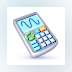# Math type 6.7 windows### MathType

MathType is an interactive equation editor that lets you create mathematical notations for word processing ...

## Programs for query  ″math type 6.7 windows″### OpenOffice.org

OpenOffice.org is an office suite designed for word processing, spreadsheets, presentations, graphics and databases.### MATLAB R2012a

MATLAB is a programming environment for algorithm development, data analysis, visualization, and numerical computation.### GeoGebra

GeoGebra is a mathematical tool that can solve equations, graph functions, create constructions, analyze data, and create 3D plots.### Microsoft Mathematics

Microsoft Mathematics provides a set of mathematical tools that help students get school work done quickly and easily.

... calculator. Additional math tools help ...### MiKTeX

MiKTeX (pronounced mick-tech) is an up-to-date implementation of TeX and related programs for Windows (all current variants).

... programs for Windows (all current ...### QM for Windows

QM for Windows provides mathematical analysis for Operations Management, Quantitative methods, or Management Science.

QM for Windows provides mathematical ...### Graph

Graph is an open source application that can be used to draw mathematical graphs in a coordinate system.### Surfer

Explore the depths of your data. Transform your data into knowledge with our affordable data modeling, mapping, graphing, and analysis software tools.